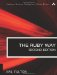# Section 2.37. Encoding and Decoding base64 Strings

### 2.36. Calculating the Levenshtein Distance Between Two Strings

The concept of distance between strings is important in inductive learning (AI), cryptography, proteins research, and in other areas.

The Levenshtein distance is the minimum number of modifications needed to change one string into another, using three basic modification operations: del(-etion), ins(-ertion), and sub(-stitution). A substitution is also considered to be a combination of a deletion and insertion (indel).

There are various approaches to this, but we will avoid getting too technical. Suffice it to say that this Ruby implementation (in Listing 2.2) allows you to provide optional parameters to set the cost for the three types of modification operations and defaults to a single indel cost basis (cost of insertion = cost of deletion).

##### Listing 2.2. The Levenshtein Distance

 `class String def levenshtein(other, ins=2, del=2, sub=1) # ins, del, sub are weighted costs return nil if self.nil? return nil if other.nil? dm = [] # distance matrix # Initialize first row values dm = (0..self.length).collect { |i| i * ins } fill =  * (self.length - 1) # Initialize first column values for i in 1..other.length dm[i] = [i * del, fill.flatten] end # populate matrix for i in 1..other.length for j in 1..self.length # critical comparison dm[i][j] = [ dm[i-1][j-1] + (self[j-1] == other[i-1] ? 0 : sub), dm[i][j-1] + ins, dm[i-1][j] + del ].min end end # The last value in matrix is the # Levenshtein distance between the strings dm[other.length][self.length] end end s1 = "ACUGAUGUGA" s2 = "AUGGAA" d1 = s1.levenshtein(s2) # 9 s3 = "pennsylvania" s4 = "pencilvaneya" d2 = s3.levenshtein(s4) # 7 s5 = "abcd" s6 = "abcd" d3 = s5.levenshtein(s6) # 0`

Now that we have the Levenshtein distance defined, it's conceivable that we could define a similar? method, giving it a threshold for similarity. For example:

`class String   def similar?(other, thresh=2)     if self.levenshtein(other) < thresh       true     else       false     end   end end if "polarity".similar?("hilarity")   puts "Electricity is funny!" end`

Of course, it would also be possible to pass in the three weighted costs to the similar? method so that they could in turn be passed into the levenshtein method. We have omitted these for simplicity.The Ruby Way, Second Edition: Solutions and Techniques in Ruby Programming (2nd Edition)
ISBN: 0672328844
EAN: 2147483647
Year: 2004
Pages: 269
Authors: Hal Fulton

Similar book on Amazon